### Entering formulas in tables

WordPerfect lets you add formulas or functions to tables. You can create formulas in tables by using arithmetic and logical operators. You can then insert the formulas in tables and floating cells. For more information about arithmetic and logical operators, see “Using operators.”

The cells in a table are labeled alphabetically from left to right and numerically from top to bottom. When you use a cell address, a comma is used to separate individual cell references; for example, if you want to add the contents of B1 and B4, you would use the formula =SUM(B1,B4). A colon is used to separate two references in a range; for example, to add all of the contents of B1 through B4 you would use =SUM(B1:B4). Table cell references can be uppercase or lowercase.

You can insert and copy formulas and functions in table cells and floating cells. You can also type formulas directly into cells if you precede the initial symbols, functions, or numbers with + or =. However, it is best to enter formulas directly only if you know how to use functions and you remember the names you want to reference in formulas. Formulas you enter are calculated automatically when you click outside the cell that contains the formula.

When you enter a formula in a table cell, an indicator will display in the lower right-hand corner of the cell. You can view the formula by pointing anywhere inside the cell. You can drag the formula indicator to another cell to copy the formula. You can also change the table settings if you do not want to show the table formula indicators.

When you no longer need a table formula, you can delete it. For information about deleting table formulas, see “To delete a table formula without deleting cell contents.” You can also protect your formulas from being deleted accidentally.

#### To enter a formula in a table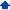1 Click Table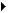Formula toolbar.
 2 Click in the Formula edit box to the right of the Accept the formula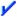button.
 3 Type cell addresses, operators, and values in the Formula edit box.
 To insert the name of a table instead of a cell address, click Names on the Table formula toolbar, click a name, and click Insert.
 4 Click one of the following:
 • Accept the formula— lets you insert the formula into the cell
 • Cancel changes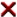— lets you cancel the formula or changes you have made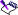• WordPerfect does not recognize formulas in cells in which the numeric format is set to Text.
 • When you insert or cancel a formula, the Formula edit mode is disabled.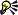• You can also click the Formula toolbar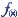button on the property bar to display the Table formula toolbar.

#### To create a function1 Click TableFormula toolbar.
 2 Click in the cell in which you want to place the function.
 3 Click the Functions button on the Table formula toolbar.
 4 Choose a function type from the Type of functions to list list box.
 5 Choose a specific function from the Functions list.
 A description of each function displays in the section below the Type of functions to list and Functions lists.
 6 Click Insert.
 7 Type cell addresses, operators, and values of the function in the Formula edit box.
 If you want to nest functions, type in the values of the initial function in the Formula edit box, and do the following:
 • Click the Functions button on the Table formula toolbar.
 • Choose a function type from the Type of functions to list list box.
 • Choose a function from the Functions list.
 A nested function can be placed in any location within the previous function.
 • Type cell addresses, operators, and values of the nested function in the Formula edit box.• In the Table functions dialog box, you can type the first few letters of a function to select it quickly.

#### To copy a table formula1 Click in the cell that contains the formula you want to copy.
 2 Click TableCopy formula.
 3 Enable one of the following options:
 • To cell — lets you type the name of the cell in which you want to paste the formula
 • Down — lets you choose the direction from the list box, and type the number of times to paste the formula up, down, left, or right• You can also click the Copy formula button on the Table formula toolbar. To display the Table formula toolbar, click TableFormula toolbar.
 • You can also copy a table formula by dragging the table formula indicator from one cell to another.

#### To disable the table formula indicators1 Click ToolsSettings.
 2 Click Display.
 3 Click the Document tab.
 4 In the Show area, disable the Table formula indicators check box.• By default, the Table formula indicators box is enabled.

#### To protect table formulas1 Click ToolsSettings.
 2 Click Environment.
 3 Click the Prompts tab.
 4 Enable the Confirm deletion of table formulas check box.